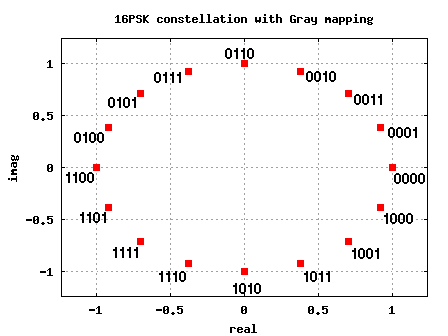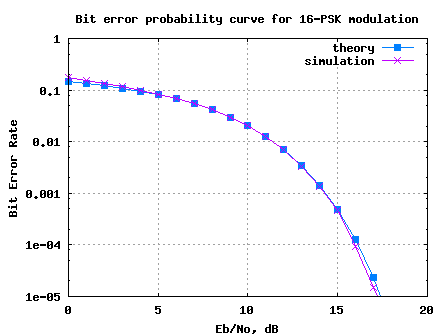# Bit error rate for 16PSK modulation using Gray mappingIn this post, let us derive the theoretical bit error probability for 16PSK modulation using Gray coded mapping. For deriving the equation, we will refer material from the following posts:

As discussed in the previous posts, the key feature of Gray code is that adjacent symbols differ by only one bit. The 16PSK constellation with Gray mapping can be as shown in the figure below.Figure: 16PSK constellation plot with Gray coded bit-mapping

From the discussion on symbol error rate for 16PSK in AWGN, we have proved that the symbol error rate for an M-PSK modulation is,

.

## Relation between bit energy Eb/No to symbol energy Es/No

As can be seen from the constellation plot, for a 16PSK modulation, each symbol transmits bits. In general, for an M-PSK modulation the number bits in each constellation symbol is,

.

Since each symbol consists of bits, the symbol to noise ratio is times the bit to noise ratio , ie.

.

## Relation between symbol error and bit error

For reasonable symbol to noise ratio , the symbol will be in error when noise causes the symbol to fall in the adjacent symbol bin. Now thanks to the Gray coded mapping, even if the symbol goes into the adjacent symbol there will be only 1 bit in error from the bits. So, the relation between symbol error and bit error is,

.

Note:

For very low symbol to noise ratio, the noise can cause the symbol to fall into the non-adjacent symbol bin. This may cause more than 1 bit error for each symbol error. However, we can ignore this case considering that we are interested only in reasonably high values of symbol to noise ratio. 🙂

## Probability of bit error for M-PSK modulation

Combining the above two equations, the bit error probability for a general M-PSK modulation is,

.

(Of course for 16-PSK, M=16).

## Matlab/Octave script for simulation 16-PSK BER

The Matlab/Octave script performs the following:

(a) Generation of random 1’s and 0’s

(b) Assigning group of 4 bits to each constellation symbol per the Gray mapping

(c) Addition of white Gaussian Noise

(d) Demodulation of PSK symbols and

(e) De-mapping per decimal to Gray conversion

(f) Counting the number of bit errors

(g) Running this for each value of Eb/No in steps of 1dB.Figure: Bit error rate plot for 16PSK modulation using Gray mapping

Observations

1. The simulated results show good agreement with the theoretical results.

2. For low Eb/No values, we can see that the simulated bit error rate is slightly higher than that expected by theory. As explained above, this can be attributed to noise causing symbol to fall into the non-adjacent bin.

Hope this helps,
Krishna

## 40 thoughts on “Bit error rate for 16PSK modulation using Gray mapping”

1.khushi says:

sir please explain Gray coded constellation mapping part in this script

1.Krishna Sankar says:
2.norseen says:

dear sir,
i want
theoryBer_16QAM

3.Mohammed says:

Yes it is throwing an error.

1.Krishna Sankar says:

@Mohammed: The script is throwing an error?

4.Mohammed says:

Hello Sir! i would really appreciate if for the 16QAM bit error rate instead of adding Gaussian noise other types of noise eg: impulsive is added.How can i do that?Pls help

1.Krishna Sankar says:

@Mohammed: You can change the noise model from AWGN.

5.shweta sharma says:

hello sir!!!!!!!!!!
plz. sir help me in finding 32 psk matlab code.

1.Krishna Sankar says:

@shweta: The script used in this post can be easily extended to 32PSK case (by changing to M=32)

1.shweta says:

thank u sir.sir if we increase the BER. The signal to noise ratio will decrease or not? plz reply…..

1.Krishna Sankar says:

@shweta: It’s the other way – a lower signal to noise ratio will cause higher bit error rate.

6.Deep says:

hi man how are u ?
i just want to ask that for 8-PSK is the same for 16-PSK and the only difference is change M from 16 to 8 ???
i look forward to hear from you

regards
Deep

1.Krishna Sankar says:

@Deep: Yes, changing 16 to 8 should suffice.

7.omer says:

hello:
i’d like to order anything about “The decorrelating detector”
thnx.

1.Krishna Sankar says:

@omer: Are you referring to decorrelating detector used in spread spectrum cases? Sorry, I do not have articles on that.

8.maytham says:

dear krishna….
iam working on ofdm system with rayleigh channel multipath using jakes model and the syntax that is availanle in matlab ‘chan=rayleighchan(ts,fd,tau,gaindb)’ but i face aproblem that when i send the data bits through this channel with three paths i always get bit error rate equal to 0.5 or little less so iam asking you if could help me to exceed this problem pls…send me the answer if you could on email…
thanks…

1.Krishna Sankar says:

@maytham: Are you equalizing for the effect of the channel in receiver?

9.Mahadevan Srinivasan says:

Hi,

I have a question on the part “Relation between symbol error and bit error”. I have read in many books and know for a fact that Pb = Ps / k, but I have not heard a reasonable explanation for that fact. Could you elaborate?

10.brunx says:

Sir,
When 16PSK is to convert into passband, how can I recover the phase in the demodulator stage? Do you know some method or equation in order to simulate it?

Thanks again, sir.

11.brunx says:

Sir,
Is it possible that the modulated signal from this simulation can be viewed in time domain? As if your looking it in the oscilloscope.

Thank you sir

12.rashed says:

dear sir,
i want
1.matalb script for simulating BER for QPSK modulation in AWGN chnanel.
2.matlab script for simulating BER for 16QAM modulation in AWGN chnanel.
3.Matlab script for simulating BER for 64QAM modulation in AWGN chnanel.
could u help me please i will very greatful to you.

1.Krishna Sankar says:
13.Ruby says:

Hi sir,
i want matlab script for comparing bit error probabilities of the 16 QAM and 16 PSK schemes using OFDM.
i will be very thankful to u.

14.Ruby says:

Hi sir,
i want matlab script for comparing bit error probabilities of the 16 QAM and 16 PSK schemes using OFDM.

15.shafqat abbas says:

i need the matlab code of coherent detection multiple phase shift keying

16.gokalp says:

hi..ı need QPSK modulatıon with gray mapping technique as a matlab code immediatelly.if you have please post ıt.thank u

1.Krishna Sankar says:

@gokalp: Sorry, I do not have posts on BER for QPSK with Gray mapping. But, it should be reasonably straightforward to scale down 16QAM BER with Gray mapping to the 4QAM case
https://dsplog.com/2008/06/05/16qam-bit-error-gray-mapping/

Good luck.

17.BusyWasp says:

Hi,
i wana matlab script for comparing bit error probabilities of the 64 QAM and 64 PSK schemes, plz?

18.Iqtadar hussain says:

sir i need matlab script for hamming code (Bit error rate plot for hamming code)

19.sumit says:

hello,
sorry i find that.

20.sumit says:

hello,
where is matlab script for psk-16 with gray code? I found here only psk-16 without gray code. but i need that. can u post that?

1.Krishna Sankar says:

@sumit: Hmm.. 🙂 Why do you say that this post discuss about BER for 16PSK without gray code?